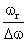NEET  >  Test: Resonance in LCR Circuit

# Test: Resonance in LCR Circuit

Test Description

## 10 Questions MCQ Test Physics For JEE | Test: Resonance in LCR Circuit

Test: Resonance in LCR Circuit for NEET 2022 is part of Physics For JEE preparation. The Test: Resonance in LCR Circuit questions and answers have been prepared according to the NEET exam syllabus.The Test: Resonance in LCR Circuit MCQs are made for NEET 2022 Exam. Find important definitions, questions, notes, meanings, examples, exercises, MCQs and online tests for Test: Resonance in LCR Circuit below.
Solutions of Test: Resonance in LCR Circuit questions in English are available as part of our Physics For JEE for NEET & Test: Resonance in LCR Circuit solutions in Hindi for Physics For JEE course. Download more important topics, notes, lectures and mock test series for NEET Exam by signing up for free. Attempt Test: Resonance in LCR Circuit | 10 questions in 10 minutes | Mock test for NEET preparation | Free important questions MCQ to study Physics For JEE for NEET Exam | Download free PDF with solutions
 1 Crore+ students have signed up on EduRev. Have you?
Test: Resonance in LCR Circuit - Question 1

### A pure resistance R, pure capacitance C and pure inductance L are connected in series and the impedance of the circuit at resonance is Z0. If they are connected in parallel to each other, the maximum impedance at resonance will be:​

Detailed Solution for Test: Resonance in LCR Circuit - Question 1

If series LCR circuit is present then z={R2+(xl-xc)2}1/2
if resonance is present then XC=CL or VC=CL then Z= Zo
if LCR circuit is in parallel form then in which circuit resonance is also present firstly 1/Z ={(1/R)2+ ((1/xc) –(1/xl))2}1/2 and in resonance conditions XC=CL according to this Z= Zo then option C is the correct answer

Test: Resonance in LCR Circuit - Question 2

### At resonance the impedance of RLC circuit is

Detailed Solution for Test: Resonance in LCR Circuit - Question 2

Resonance is a condition in LCR circuit where the inductive reactance ( XL) and capacitive reactance (Xc) is equal.
Impedance at resonance is equal to the Resistance of the resistor because the other two cancel themselves off according to the below formula -
Z2=R2+(XL2-Xc2)
As XL = Xc ,
Z = R

Test: Resonance in LCR Circuit - Question 3

### Ratiois equal to

Detailed Solution for Test: Resonance in LCR Circuit - Question 3

frequency-to-bandwidth ratio of the resonator:
Q=fr/△f= ωr/△ω
where fr is the resonant frequency, Δf is the resonance width or full width at half maximum (FWHM) i.e. the bandwidth over which the power of vibration is greater than half the power at the resonant frequency, ωr = 2πfr is the angular resonant frequency, and Δω is the angular half-power bandwidth.

Test: Resonance in LCR Circuit - Question 4

When the oscillations produced are of constant amplitude. The are called

Detailed Solution for Test: Resonance in LCR Circuit - Question 4

If the losses incurred in the electrical system could be compensated, the amplitude of oscillation would remain constant and as such the oscillation would continue indefinitely against both external disturbances and changes in the initial conditions. This type of oscillation is called undamped oscillation.

Test: Resonance in LCR Circuit - Question 5

Find the series resonance frequency when L = 0.09 H, C = 25 F​

Detailed Solution for Test: Resonance in LCR Circuit - Question 5

ω=1/√LC
ω=1√0.09x25
ω=0.666
ω=2πf
f=0.666/(2 x 3.14)
f=0.106 s-1

Test: Resonance in LCR Circuit - Question 6

Measure of sharpness in an ac circuit is given by

Test: Resonance in LCR Circuit - Question 7

In a series RCL circuit at resonance the applied ac voltage is 220 V. The potential drop across the inductance is 110V, what is the potential drop across the capacitor?​

Detailed Solution for Test: Resonance in LCR Circuit - Question 7

At resonance, the potential drop across the capacitance is equal and opposite to that of inductance i.e. 110V.

Test: Resonance in LCR Circuit - Question 8

Acceptor circuit is

Detailed Solution for Test: Resonance in LCR Circuit - Question 8

The series resonance circuit is known as an acceptor circuit. The impedance of the acceptor circuit is minimum but the voltage can be magnified.
The Acceptor circuit provides the maximum response to currents at its resonant frequency.
Series resonance circuit is known as acceptor circuit because the impedance at the resonance is at its minimum so as to accept the current easily such that the frequency of the accepted current is equal to the resonant frequency.

Test: Resonance in LCR Circuit - Question 9

An ac circuit contains a resistance R, capacitance C and inductance L in series with a source of emf ε = ε0sin (ωt – Ø). The current through the circuit is maximum when:​

Test: Resonance in LCR Circuit - Question 10

Resonant circuits are used in tuning of

Detailed Solution for Test: Resonance in LCR Circuit - Question 10

The most common application of tank circuits is tuning radio transmitters and receivers. For example, when we tune a radio to a particular station, the LC circuits are set at resonance for that particular carrier frequency. A parallel resonant circuit can be used as load impedance in output circuits of RF amplifiers.

## Physics For JEE

199 videos|531 docs|256 tests
 Use Code STAYHOME200 and get INR 200 additional OFF Use Coupon Code
Information about Test: Resonance in LCR Circuit Page
In this test you can find the Exam questions for Test: Resonance in LCR Circuit solved & explained in the simplest way possible. Besides giving Questions and answers for Test: Resonance in LCR Circuit, EduRev gives you an ample number of Online tests for practice

## Physics For JEE

199 videos|531 docs|256 tests

### How to Prepare for NEET

Read our guide to prepare for NEET which is created by Toppers & the best Teachers